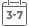# Introduction to Numerical Ordinary and Partial Differential Equations Using MATLAB

• Publish Date: 2004-12-31
• Binding: Hardcover
• Author: Alexander Stanoyevitch
• Sale
• \$28.89
• Regular price \$212.91Learn how to solve complex differential equations using MATLAB

Introduction to Numerical Ordinary and Partial Differential Equations Using MATLAB teaches readers how to numerically solve both ordinary and partial differential equations with ease. This innovative publication brings together a skillful treatment of MATLAB and programming alongside theory and modeling. By presenting these topics in tandem, the author enables and encourages readers to perform their own computer experiments, leading them to a more profound understanding of differential equations.

The text consists of three parts:

• Introduction to MATLAB and numerical preliminaries, which introduces readers to the software and itsgraphical capabilities and shows how to use it to write programs
• Ordinary Differential Equations
• Partial Differential Equations

All the tools needed to master using MATLAB to solve differential equations are provided and include:

• Exercises for the Reader that range from routine computations to more advanced conceptual and theoretical questions (solutions appendix included)
• Illustrative examples, provided throughout the text, that demonstrate MATLAB's powerful ability to solve differential equations
• Explanations that are rigorous, yet written in a very accessible, user-friendly style